### [翻译]]ARM汇编简介（四）载入/存储多个值，入栈和出栈

2018-6-28 16:36
3611

Sometimes it is more efficient to load (or store) multiple values at once. For that purpose we use LDM (load multiple) and STM (store multiple). These instructions have variations which basically differ only by the way the initial address is accessed. This is the code we will use in this section. We will go through each instruction step by step.

```.data

array_buff:
.word 0x00000000             /* array_buff */
.word 0x00000000             /* array_buff */
.word 0x00000000             /* array_buff. This element has a relative address of array_buff+8 这里存储了array_buff+8的相对地址*/
.word 0x00000000             /* array_buff */
.word 0x00000000             /* array_buff */

.text
.global _start

_start:
ldr r1, array_buff_bridge    /* address of array_buff -> r1  将 array_buff的地址给r1*/
ldr r2, array_buff_bridge+4  /* address of array_buff -> r2   将 array_buff的地址给r2*/
ldm r0, {r4,r5}              /* words -> r4 = 0x03; words -> r5 = 0x04  */
stm r1, {r4,r5}              /* r4 -> array_buff = 0x03; r5 -> array_buff = 0x04 */
ldmia r0, {r4-r6}            /* words -> r4 = 0x03, words -> r5 = 0x04; words -> r6 = 0x05; */
stmia r1, {r4-r6}            /* r4 -> array_buff = 0x03; r5 -> array_buff = 0x04; r6 -> array_buff = 0x05 */
ldmib r0, {r4-r6}            /* words -> r4 = 0x04; words -> r5 = 0x05; words -> r6 = 0x06 */
stmib r1, {r4-r6}            /* r4 -> array_buff = 0x04; r5 -> array_buff = 0x05; r6 -> array_buff = 0x06 */
ldmda r0, {r4-r6}            /* words -> r6 = 0x03; words -> r5 = 0x02; words -> r4 = 0x01 */
ldmdb r0, {r4-r6}            /* words -> r6 = 0x02; words -> r5 = 0x01; words -> r4 = 0x00 */
stmda r2, {r4-r6}            /* r6 -> array_buff = 0x02; r5 -> array_buff = 0x01; r4 -> array_buff = 0x00 */
stmdb r2, {r4-r5}            /* r5 -> array_buff = 0x01; r4 -> array_buff = 0x00; */
bx lr

words:
.word 0x00000000             /* words */
.word 0x00000001             /* words */
.word 0x00000002             /* words */
.word 0x00000003             /* words */
.word 0x00000004             /* words */
.word 0x00000005             /* words */
.word 0x00000006             /* words */

array_buff_bridge:
.word array_buff             /* address of array_buff, or in other words - array_buff */
.word array_buff+8           /* address of array_buff */```

Before we start, keep in mind that a .word refers to a data (memory) block of 32 bits = 4 BYTES. This is important for understanding the offsetting. So the program consists of .data section where we allocate an empty array (array_buff) having 5 elements. We will use this as a writable memory location to STORE data. The .text section contains our code with the memory operation instructions and a read-only data pool containing two labels: one for an array having 7 elements, another for “bridging” .text and .data sections so that we can access the array_buff residing in the .data section.

`adr r0, words+12             /* address of words -> r0   将word的地址传送给r0*/`

We use ADR instruction (lazy approach) to get the address of the 4th(words) element into the R0. We point to the middle of the words array because we will be operating forwards and backwards from there.

```gef> break _start
gef> run
gef> nexti```

R0 now contains the address of word, which in this case is 0x80B8. This means, our array starts at the address of word: 0x80AC (0x80B8 –  0xC).

```gef> x/7w 0x00080AC
0x80ac <words>: 0x00000000 0x00000001 0x00000002 0x00000003
0x80bc <words+16>: 0x00000004 0x00000005 0x00000006```

We prepare R1 and R2 with the addresses of the first (array_buff) and third (array_buff) elements of the array_buff array. Once the addresses are obtained, we can start operating on them.

```ldr r1, array_buff_bridge    /* address of array_buff -> r1 */
ldr r2, array_buff_bridge+4  /* address of array_buff -> r2 */```

After executing the two instructions above, R1 and R2 contain the addresses of array_buff and array_buff.

```gef> info register r1 r2   //用于获取寄存器的值
r1      0x100d0     65744
r2      0x100d8     65752```

The next instruction uses LDM to load two word values from the memory pointed by R0. So because we made R0 point to words element earlier, the words value goes to R4 and the words value goes to R5.

`ldm r0, {r4,r5}              /* words -> r4 = 0x03; words -> r5 = 0x04 */`

We loaded multiple (2 data blocks) with one command, which set R4 = 0x00000003 and R5 = 0x00000004.

```gef> info registers r4 r5
r4      0x3      3
r5      0x4      4```

So far so good. Now let’s perform the STM instruction to store multiple values to memory. The STM instruction in our code takes values (0x3 and 0x4) from registers R4 and R5 and stores these values to a memory location specified by R1. We previously set the R1 to point to the first array_buff element so after this operation the array_buff = 0x00000003 and array_buff = 0x00000004. If not specified otherwise, the LDM and STM opperate on a step of a word (32 bits = 4 byte).

`stm r1, {r4,r5}              /* r4 -> array_buff = 0x03; r5 -> array_buff = 0x04 */`

The values 0x3 and 0x4 should now be stored at the memory address 0x100D0 and 0x100D4. The following instruction inspects two words of memory at the address 0x000100D0.

```gef> x/2w 0x000100D0
0x100d0 <array_buff>:  0x3   0x4```

As mentioned before, LDM and STM have variations. The type of variation is defined by the suffix of the instruction. Suffixes used in the example are: -IA (increase after), -IB (increase before), -DA (decrease after), -DB (decrease before). These variations differ by the way how they access the memory specified by the first operand (the register storing the source or destination address). In practice, LDM is the same as LDMIA, which means that the address for the next element to be loaded is increased after each load. In this way we get a sequential (forward) data loading from the memory address specified by the first operand (register storing the source address).

```ldmia r0, {r4-r6} /* words -> r4 = 0x03, words -> r5 = 0x04; words -> r6 = 0x05;  */
stmia r1, {r4-r6} /* r4 -> array_buff = 0x03; r5 -> array_buff = 0x04; r6 -> array_buff = 0x05 */```

After executing the two instructions above, the registers R4-R6 and the memory addresses 0x000100D0, 0x000100D4, and 0x000100D8 contain the values 0x3, 0x4, and 0x5.

```gef> info registers r4 r5 r6
r4     0x3     3
r5     0x4     4
r6     0x5     5
gef> x/3w 0x000100D0
0x100d0 <array_buff>: 0x00000003  0x00000004  0x00000005```

The LDMIB instruction first increases the source address by 4 bytes (one word value) and then performs the first load. In this way we still have a sequential (forward) loading of data, but the first element is with a 4 byte offset from the source address. That’s why in our example the first element to be loaded from the memory into the R4 by LDMIB instruction is 0x00000004 (the words) and not the 0x00000003 (words) as pointed by the R0.

LDMIB指令先将源地址增加4字节（一个字），然后开始载入数据。该方法仍能让我们载入一串前向数据，但第一个数据的起始地址相较源地址有4个字节的偏移。这就是为什么我们用LDMIB指令将内存中的第一个元素载入R4后，R4是0x04（word），而不是R0指向的0x3（word）

```ldmib r0, {r4-r6}            /* words -> r4 = 0x04; words -> r5 = 0x05; words -> r6 = 0x06 */
stmib r1, {r4-r6}            /* r4 -> array_buff = 0x04; r5 -> array_buff = 0x05; r6 -> array_buff = 0x06 */```

After executing the two instructions above, the registers R4-R6 and the memory addresses 0x100D4, 0x100D8, and 0x100DC contain the values 0x4, 0x5, and 0x6.

```gef> x/3w 0x100D4
0x100d4 <array_buff+4>: 0x00000004  0x00000005  0x00000006
gef> info register r4 r5 r6
r4     0x4    4
r5     0x5    5
r6     0x6    6```

`ldmda r0, {r4-r6} /* words -> r6 = 0x03; words -> r5 = 0x02; words -> r4 = 0x01 */`

Registers R4, R5, and R6 after execution:

R4，R5，R6的变化：

```gef> info register r4 r5 r6
r4     0x1    1
r5     0x2    2
r6     0x3    3```

`ldmdb r0, {r4-r6} /* words -> r6 = 0x02; words -> r5 = 0x01; words -> r4 = 0x00 */`

Registers R4, R5, and R6 after execution:

R4，R5，R6的变化

```gef> info register r4 r5 r6
r4 0x0 0
r5 0x1 1
r6 0x2 2```

Store multiple, decrement after.

`stmda r2, {r4-r6} /* r6 -> array_buff = 0x02; r5 -> array_buff = 0x01; r4 -> array_buff = 0x00 */`

Memory addresses of array_buff, array_buff, and array_buff after execution:

```gef> x/3w 0x100D0
0x100d0 <array_buff>: 0x00000000 0x00000001 0x00000002```

Store multiple, decrement before:

`stmdb r2, {r4-r5} /* r5 -> array_buff = 0x01; r4 -> array_buff = 0x00; */`

Memory addresses of array_buff and array_buff after execution:

```gef> x/2w 0x100D0
0x100d0 <array_buff>: 0x00000000 0x00000001```

# PUSH AND POP  push和pop

There is a memory location within the process called Stack. The Stack Pointer (SP) is a register which, under normal circumstances, will always point to an address wihin the Stack’s memory region. Applications often use Stack for temporary data storage. And As mentioned before, ARM uses a Load/Store model for memory access, which means that the instructions LDR / STR or their derivatives (LDM.. /STM..) are used for memory operations. In x86, we use PUSH and POP to load and store from and onto the Stack. In ARM, we can use these two instructions too:

When we PUSH something onto the Full Descending (more about Stack differences inPart 7: Stack and Functions) stack the following happens:

1.First, the address in SP gets DECREASED by 4.

2.Second, information gets stored to the new address pointed by SP.

When we POP something off the stack, the following happens:

1.The value at the current SP address is loaded into a certain register,

2.Address in SP gets INCREASED by 4.

SP里的值加4

In the following example we use both PUSH/POP and LDMIA/STMDB:

```.text
.global _start

_start:
mov r0, #3
mov r1, #4
push {r0, r1}
pop {r2, r3}
stmdb sp!, {r0, r1}
ldmia sp!, {r4, r5}
bkpt```

Let’s look at the disassembly of this code. 看看汇编代码是什么样的

```azeria@labs:~\$ as pushpop.s -o pushpop.o
azeria@labs:~\$ ld pushpop.o -o pushpop
azeria@labs:~\$ objdump -D pushpop
pushpop: file format elf32-littlearm

Disassembly of section .text:

00008054 <_start>:
8054: e3a00003 mov r0, #3
8058: e3a01004 mov r1, #4
805c: e92d0003 push {r0, r1}
8060: e8bd000c pop {r2, r3}
8064: e92d0003 push {r0, r1}
8068: e8bd0030 pop {r4, r5}
806c: e1200070 bkpt 0x0000```

As you can see, our LDMIA and STMDB instuctions got translated to PUSH and POP. That’s because PUSH is a synonym for STMDB sp!, reglist and POP is a synonym for LDMIA sp! reglist (seeARM Manual)

Let’s run this code in GDB.在GDB里运行一下

```gef> break _start
gef> run
gef> nexti 2
[...]
gef> x/w \$sp
0xbefff7e0: 0x00000001```

After running the first two instructions we quickly checked what memory address and value SP points to. The next PUSH instruction should decrease SP by 8, and store the value of R1 and R0 (in that order) onto the Stack.

```gef> nexti
[...] ----- Stack -----
0xbefff7d8|+0x00: 0x3 <- \$sp
0xbefff7dc|+0x04: 0x4
0xbefff7e0|+0x08: 0x1
[...]
gef> x/w \$sp
0xbefff7d8: 0x00000003```

Next, these two values (0x3 and 0x4) are poped off the Stack into the registers, so that R2 = 0x3 and R3 = 0x4. SP is increased by 8:

```gef> nexti
gef> info register r2 r3
r2     0x3    3
r3     0x4    4
gef> x/w \$sp
0xbefff7e0: 0x00000001```

[2023春季班]2023，新的征程，脱壳机更新、iOS/eBPF、赠送云手机套装！一块裸板虚拟化五个容器云手机！3月25日起同时上调价格并赠送新设备！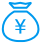打赏次数 1 雪花 + 5.00junkboy 5 2018/06/28hasking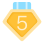2018-6-28 16:56
2
0MaYil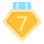2018-6-28 18:29
3
0junkboy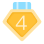2018-6-28 21:35
4
0r0Cat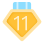7 2018-6-28 23:02
5
0junkboy 看完啦 谢谢翻译 希望楼主心情一直好 等下一集～